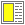# Exercise solution: Using flip, negate, and compose

I would try the following activations of flip, negate and compose.The notation expr => v means that the expression gives the value v when evaluated by Scheme.

1. ((flip -) 5 6)   =>   1
2. ((flip cons) 'a 'b)   =>   (b . a)
3. (let ((non-string? (negate string?))) (non-string? 5))   =>   #t
4. ((compose b em) "bold emphasize text")   =>   "<b><em>bold emphasize text</em></b>"